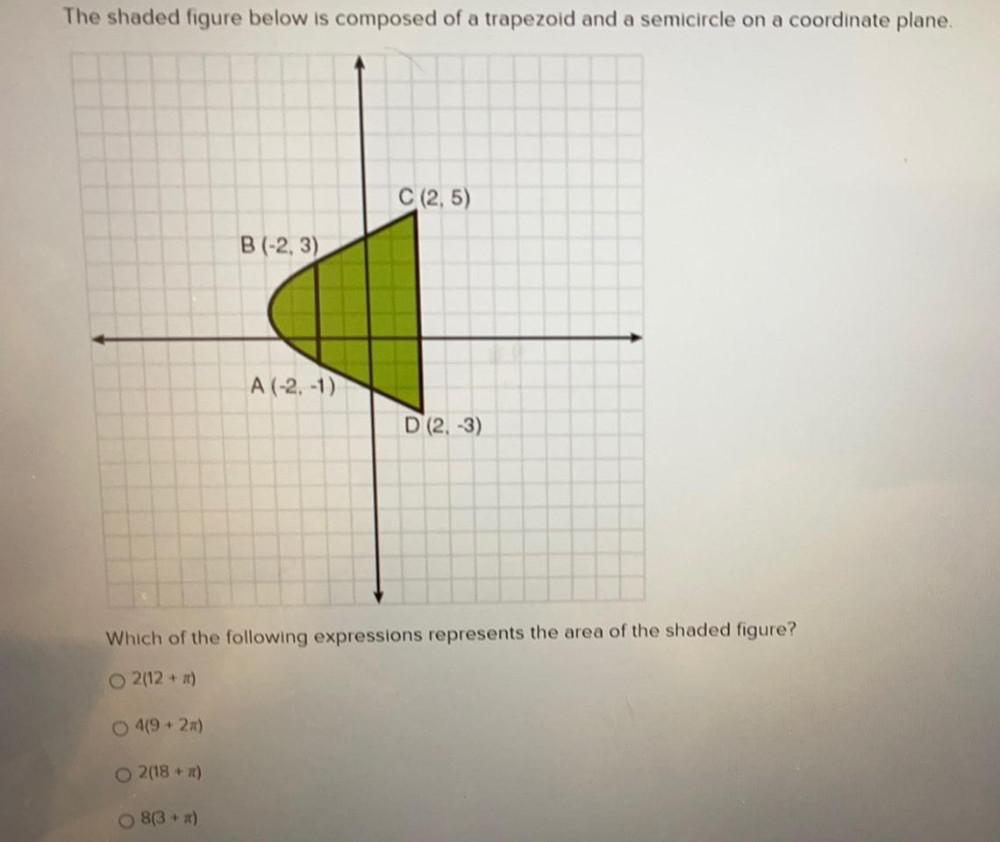Question:

# The shaded figure below is composed of a trapezoid and a semicircle on a coordinate plane. C (2,5) B (-2, 3) A (-2,-1) D (2-3) WThe shaded figure below is composed of a trapezoid and a semicircle on a coordinate plane. C (2,5) B (-2, 3) A (-2,-1) D (2-3) Which of the following expressions represents the area of the shaded figure? O2(12 + m) 0 419 + 2x) o 2(18+) 8(3+)